Friday, February 5, 2016

Decoupling RF Circuits - Part 1

Several weeks ago I saw a RF circuit that scared me from the “Measurement Repeatability” standpoint. The circuit in question had RF at one end and DC at the other, simple enough and any number of modern devices contain such circuits. Whether it be a Bluetooth module or a PLL Synthesizer IC, a simple RF switch or as in this case one of those very useful little RF Power to DC converter IC's.

The design intent here was to make a decent RF power measurement at the input to the circuit and then digitize the output and pass that result on to a controller. The design was straight forward enough. The board was laid out with a reasonable SMA edge launch connector and passable RF trace design to the IC. What caught my eye however was the DC portion of the circuit layout. This should have been the easy part, but as it turns out the physical implementation of the DC circuit had a very large impact on the circuits RF performance.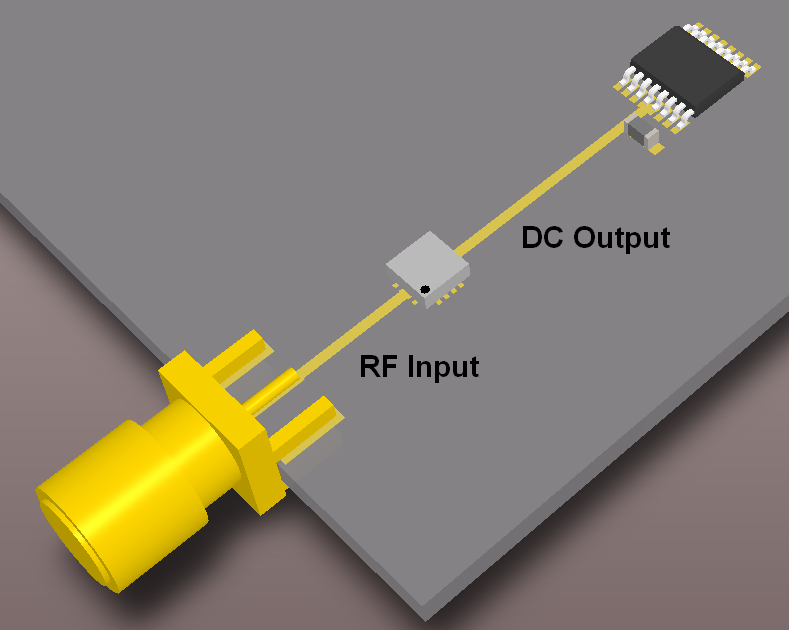Figure 1 – A simplified representation of the physical layout is shown above, straightforward enough, but as it turns out the DC portion of this circuit had a very large impact on its RF performance of this particular circuit.

The DC output trace looked exactly like the RF input trace. Now the RF input trace would be properly terminated by whatever is connected to the SMA input one end and by the IC + Matching network on the other end. If you remember your transmission line theory a matched transmission line will have a low VSWR (also known as low reflection) and exhibit very little amplitude ripple due to mismatch and hence will have low measurement uncertainty.

An improperly matched transmission line will act like a resonant structure with the potential for an amazingly large Q. The DC output trace was clearly the same width as the RF input trace, it was also longer than the RF input trace (about 0.6” long). Since the operating frequency range of the circuit was upwards of 10 GHz, this means that the supposedly DC output trace would will likely resonate in the band of operation. Schematically the equivalent circuit could be modeled as in Figure 2.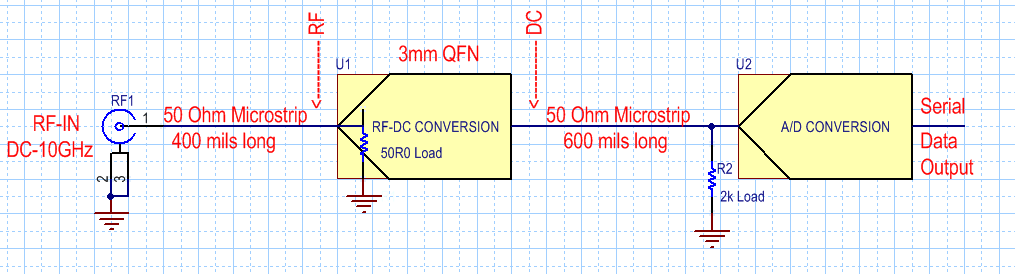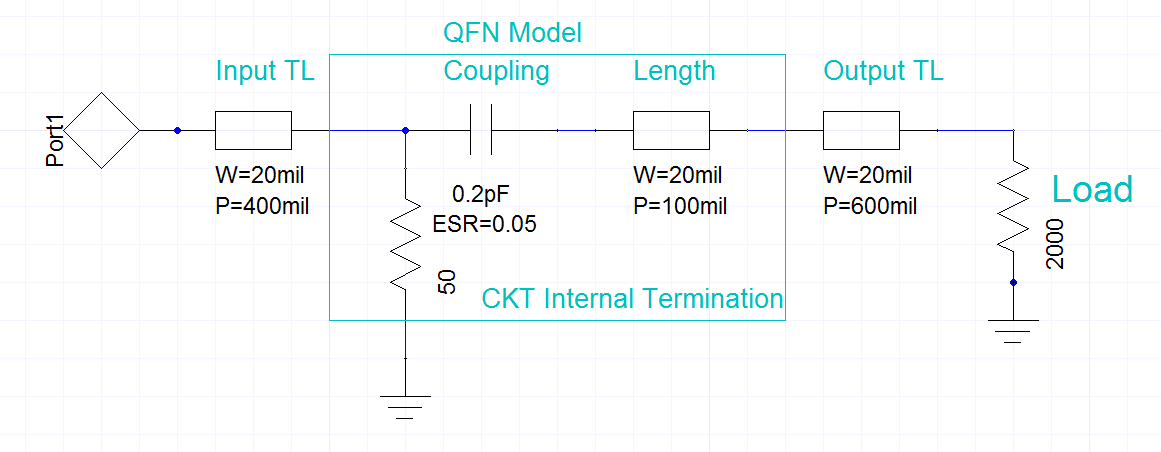Figure 2 – Above is a schematic representation of the circuit and below is the simplified transmission line equivalent. Estimating 0.1 to 0.2 pF of coupling across a small IC die like this seems entirely likely and at high frequency’s will certainly be a significant amount of coupling. The 3mm QFN also adds effective length to the circuit so it has to be taken into to account also if one wants to simulate the resonance peaks at the proper frequencies.

I Hooked the circuit board up to my network analyzer and measured the S11 and found the expected result. The input match was not well controlled and did indeed show all the characteristics of coupling to a high Q structure (Figure 3).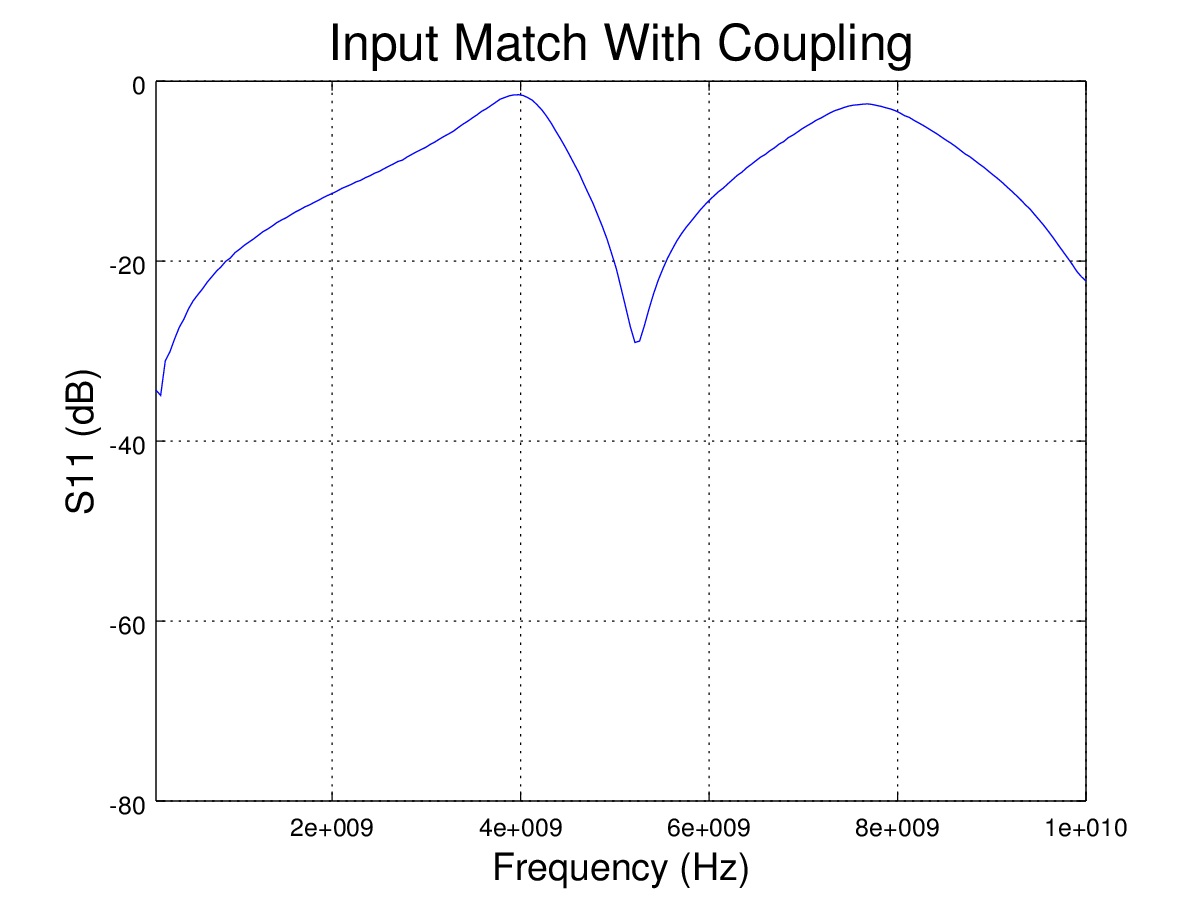Figure 3 – The actual measured S11 (Input Match) to the circuit showed the expected and undesired coupling. The actual measured S11 was less than -5dB in a couple of spots. That poor of a match (under 3 dB!) can add quite a bit of amplitude uncertainty to any measurement.

To make sure that the coupling was indeed caused by the DC Output trace and not some other problem, I removed the QFN and replaced it by using two 100 ohm 0402 resistors connected in parallel to the ground plane on either side of the RF input trace directly at the QFN Pads, this gives a very low inductance and capacitance 50 ohm load to analyze just the RF input trace, I also modified the input matching to account for this. The results of just measuring the RF input trace are shown in figure 4.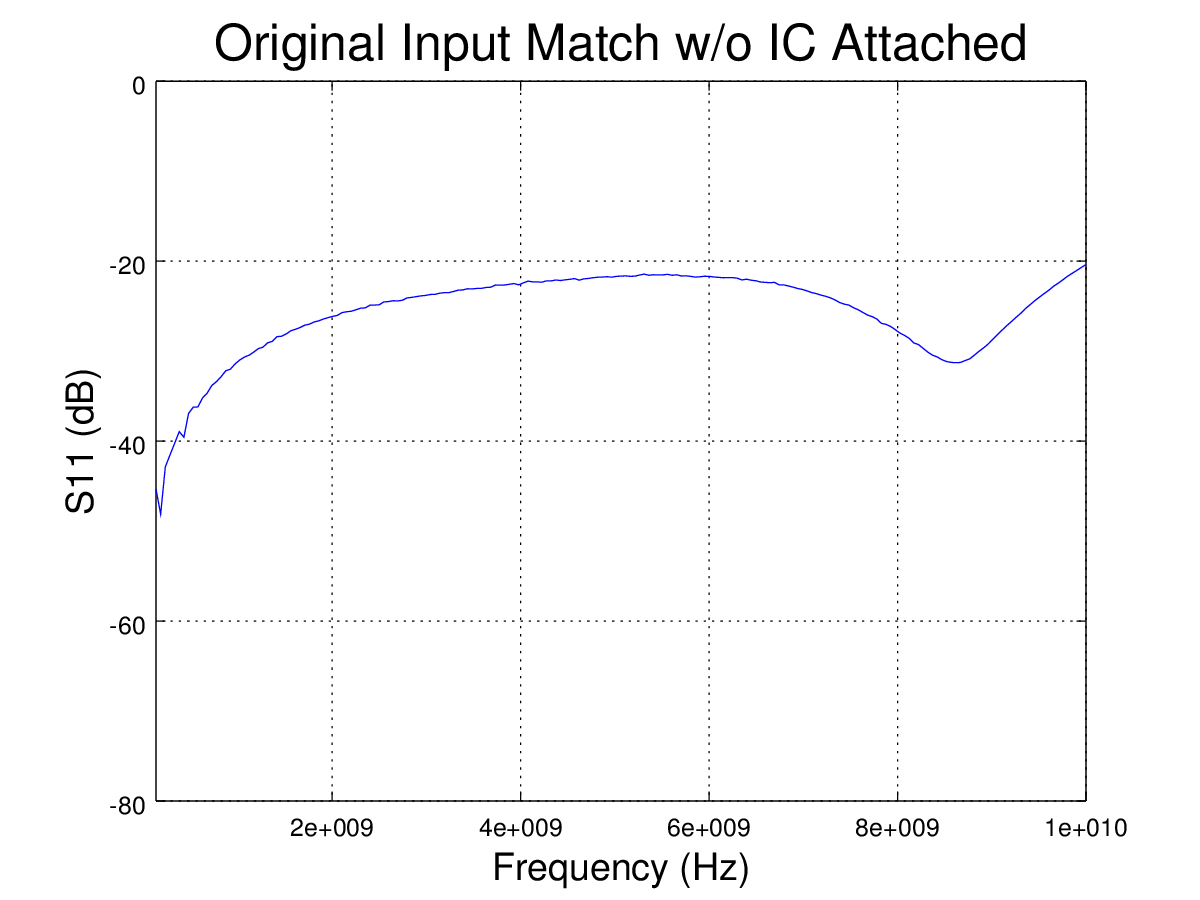Figure 4 – Repeating the S11 measurement with the IC replaced by two 100 Ohm 0402 resistors in parallel directly at the QFN input pins shows that the RF portion of the circuit was quite well behaved and actually had an outstanding match all the way up to 10 GHz.

Repeating the measurement a third time with just a 0.2 pF capacitor bridged across the RF trace to the DC output showed essentially the same results as figure 3, thus confirming the cause of the bad match as the DC output trace resonating. A simulation of the transmission line equivalent circuit of Figure 2B also showed about the same response as the actual measurements as is shown in Figure 5.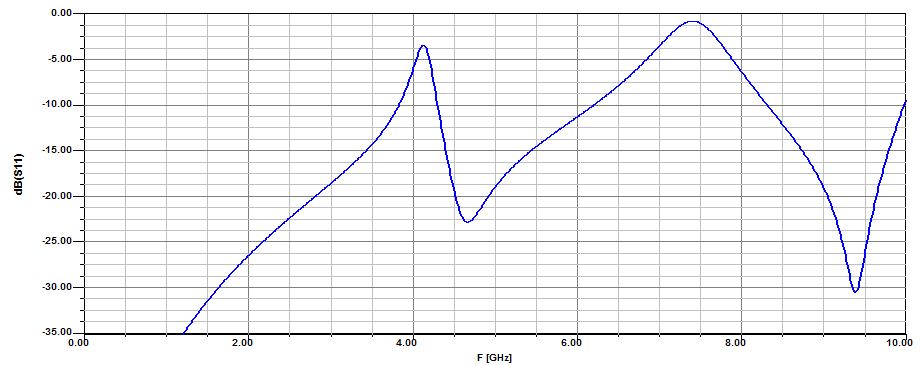Figure 5 – Simulating the equivalent circuit of Figure 2B and we see essentially the same as the measured response, the first peak is at about 4 GHz as was measured. The continued sharp resonances in the simulation are because this simple linear simulation does not include real PCB and circuit losses, hence the simulated Q's are higher than actual.

Decoupling Is The Solution

The basic solution to this problem is to decouple the DC output trace properly.

Step #1 When designing the PCB in the first place, narrow the trace to a more reasonable width for a DC signal, narrowing this trace from 20 mils to 6 mils changes it's impedance from 50 ohms to around 95 ohms. There was simply no reason to have the DC trace the width of a 50 Ohm line other that it: “Looked Pretty”. By making the trace narrower it will have more loss at high frequencies and when it is a higher impedance, it will be easier to decouple.

Step #2 Is to keep the RF off the trace as best we can in the first place. This can be accomplished by decoupling the DC output from the trace. This solution can also be hacked on an already built PCB as a band-aid.

Decoupling essentially puts some sort of low pass filter between the IC and the output trace to keep the RF off the trace in the first place. This can be accomplished many ways here I will present one of my favorites, simple RC filtering.

By adding a simple and low cost RC Low Pass Filter as close as possible to the IC (Figure 6), RF can effectively kept off the output trace really reducing coupling.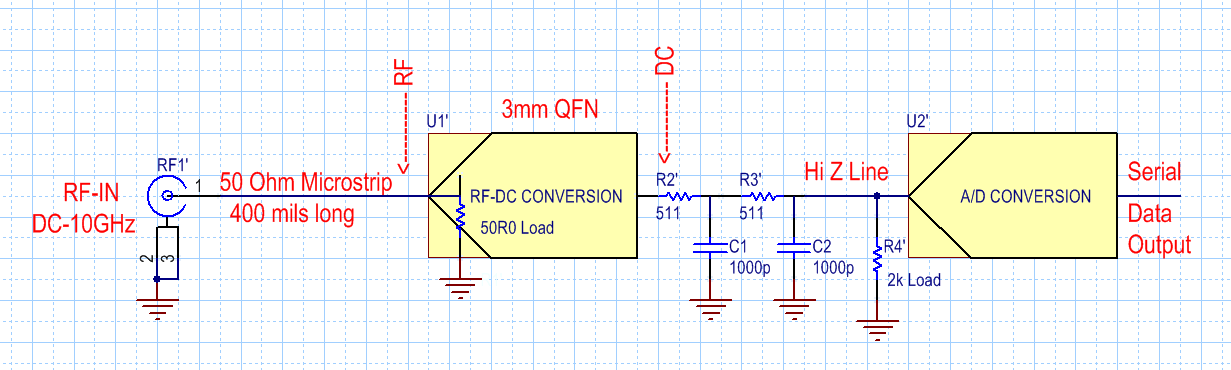Figure 6 – Modifying the original circuit by adding R2, R3, C1 & C2 to decouple the RF energy at the IC from getting on the long output trace and causing resonances can be accomplished by one of my favorite techniques shown above. I size the resistors and capacitors based on the technology that the majority of the rest of the design is using, normally this means either 0603 or 0402 sized parts.

Figure 7 shows the measurement results of this simple addition when it was hacked on to the test PCB.

In circuits like Figure 6 shows I usually start with 200 to 511 Ohm resistors as they are relatively flat over a large frequency range such as this and the largest COG capacitor I can find in whatever size the design is using, in this case it was a 1000 pF / 0603 Sized part. COG Temperature coefficient parts typically have better RF Performance because of lower losses.

The circuit of Figure 6 also adds a voltage divider that must be taken into account in the overall final design, in this case the A/D's gain was adjusted to compensate for the extra drop across the two 511 ohm resistors. Simulation also showed that the resistors can be made about 200 ohms each with nearly the same decoupling result.

Of note: Normally we would like to add a capacitor directly at every DC pin on the IC, but this particular pin is an OPAMP output and we don't want to make the OPAMP unstable with the addition of a capacitor directly at it's output.

Finally to test the decoupling circuit itself to make sure that no unruly resonances are occurring there, I connected SMA cables to both sides of the DC output trace and did a S21 through line measurement. The results shown in figure 8 show a nicely damped response with no funny resonances.

Variations on a Theme

There are naturally as many possible variations on making a suitable decoupling network as there are designers and there are many different circuit requirements too. For instance, the RC decoupling circuit of Figure 6 would not work very well for a DC bias line as it would produce a lot of voltage drop when the current get's into the MilliAmp range. Likewise the 3dB bandwidth of Figure 6 is just a little over 100 kHz, so using this decoupling network on a I2C or SPI line won't work very well either unless the clock frequency is very low. So there are many requirements to consider before selecting the actual circuit values.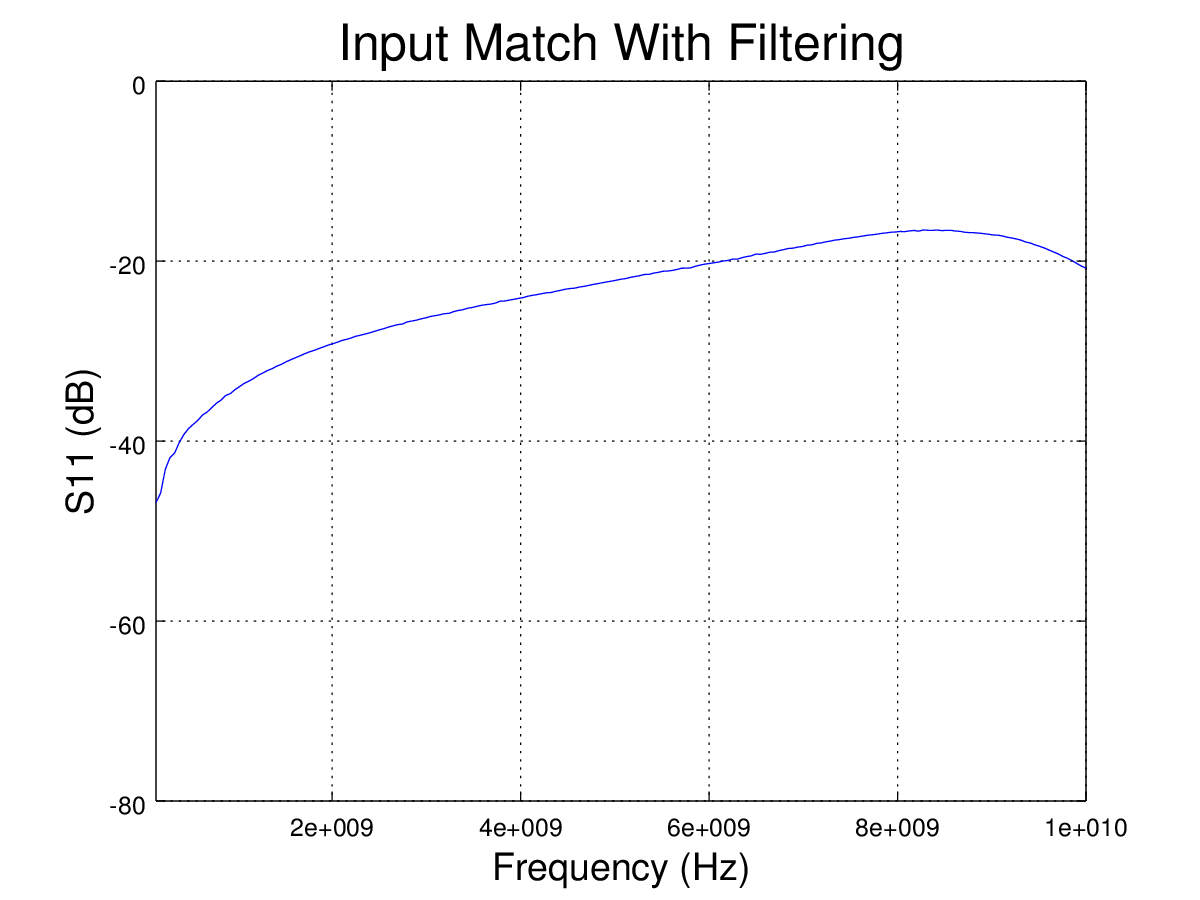Figure 7 – Adding Figure 6's decoupling to the original circuit tamed resonance on that long and wide DC trace to a very reasonable level, there is still coupling but the input Match is much more well behaved (Better than 17 dB everywhere). All this without even touching the actual input trace or match at all.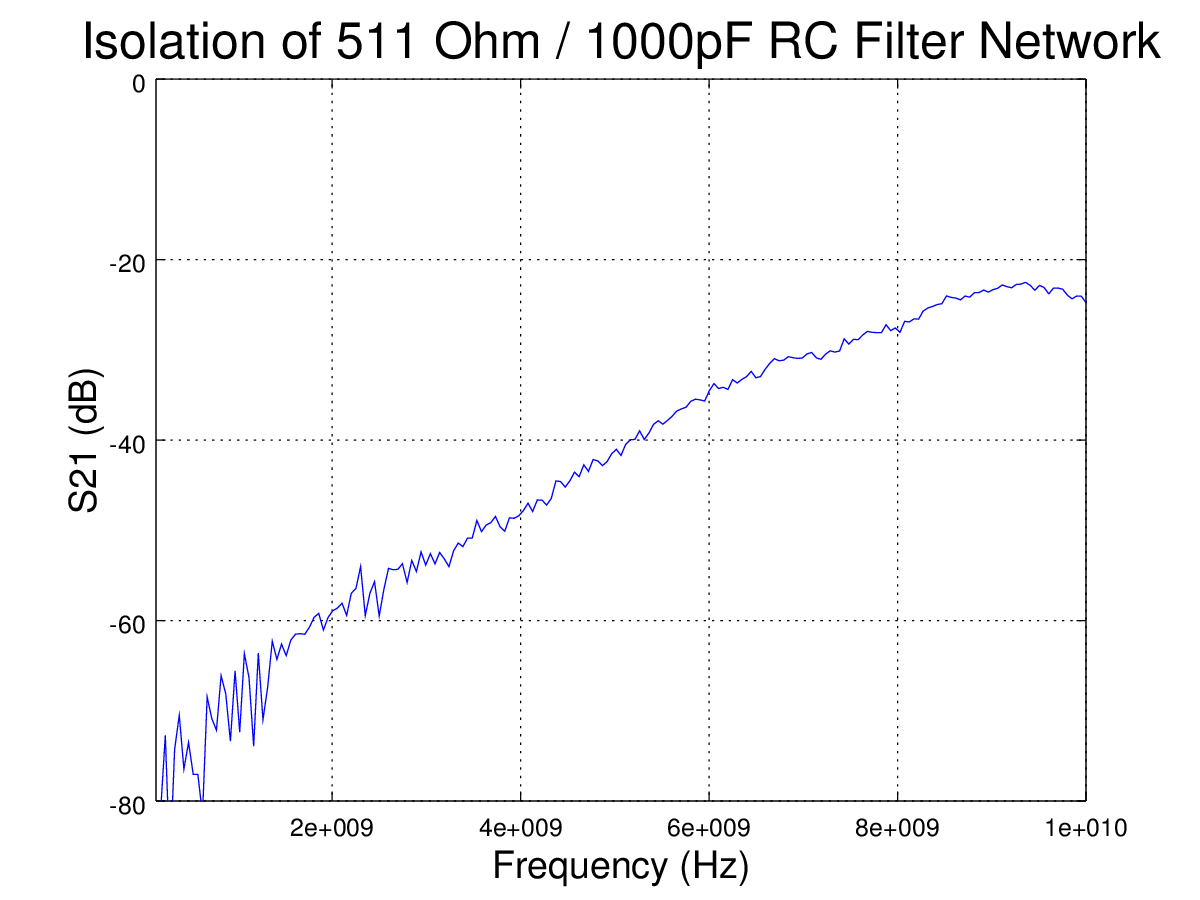Figure 8 – S21 Measurement of the through response from one side of the long DC trace to the other shows a nicely damped response with no troublesome resonances anywhere. The reason the response is rising instead of falling is because at high frequencies the 1000 pF decoupling capacitors are actually inductive because of the package parasitics. 0402 Sized parts have less package inductance and for higher frequency circuits may be more appropriate.

Appendix: Sometimes finding the culprit resonance is not so easy

Sometimes it is not clear on a complex PCB what is causing the problem resonances, in these cases a bit of detective work is required. Remember that a poorly terminated transmission line will resonate when the frequency reaches a quarter wavelength and every quarter wavelength after that it will resonate with the opposite impedance.

Start off by measuring the resonant peak frequency or if multiple peaks, the delta frequency between peaks, then calculate the transmission line length that will give these frequency peaks and start looking for trace lengths on the PCB that match the calculation and pretty rapidly you will find the culprit. Sometimes you can also use the "Move your finger around touching things" method.

If your frequency sweep is from a low frequency and your circuit is fine, then suddenly there is a resonance, you are probably seeing a first quarter wavelength effect (Also see Reference ).

On the other hand if you are sweeping across some very high frequency band and you see some repeating ripple pattern then you are probably seeing a one half wavelength effect. At every half wavelength the impedance of "whatever" that is resonating will circle around to the same impedance again, hence giving rise to a repeating pattern.

In practice I have found problems in the range of 7 inches for a cable that ran off the PCB and then back on again causing a resonance to traces like this example PC that were a few tenths of an inch long.

Some common approximate frequencies and quarter wavelength for Microstrip on FR4 are given in the table below,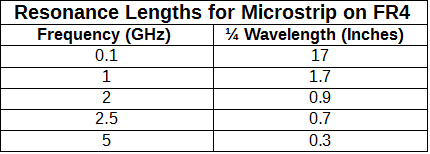The Table can be written as a formula like,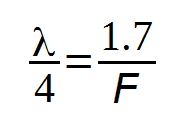-
Where the Microstrip quarter wavelength on FR4 is in inches and F is the frequency in GHz.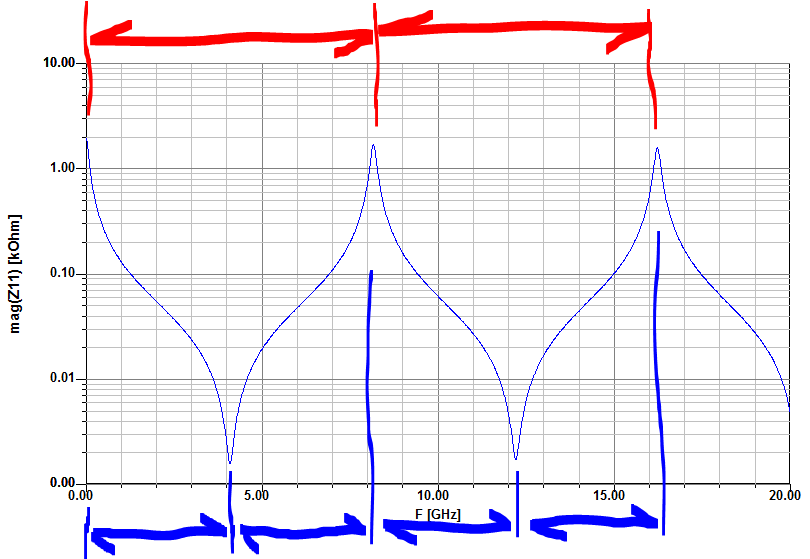Figure A.1 – As an example of the kind of repeating pattern that can be seen on a transmission line. Here an open circuited line was simulated. The Y axis here is Impedance Magnitude in Ohms and the X Axis is frequency.

The blue arrows on the bottom mark off ¼ wavelengths. As can be seen, a open circuit transmission line resonates every ¼ wavelength but the impedance at each ¼ wavelength goes from high impedance to low impedance and back again.

The red arrows at the top mark off ½ wavelength segments. On this open circuited line the ½ wavelength repeating pattern is at a very high impedance and there is also a repeating ½ wavelength pattern at low impedances (the peaks on the bottom of the graph, not marked).

As a note, a short circuited line has exactly the same pattern, it is just reversed in impedance. That is, instead of starting at a high impedance at low frequencies, it starts at a low impedance and the first ¼ resonant peak is at a high impedance, not the low impedance shown here. Then the pattern repeats the same as for this simulation.

The simulated response shown in Figure A.1 is for some randomly selected line length. Note that a longer line would produce peaks closer together while a shorter line would produce peaks that are farther apart.

References:

 A resonance effect on a RF circuit can also be caused by any shielding or box that the circuit is put into. You can read more about that here,

By: Steve Hageman www.AnalogHome.com

We design custom: Analog, RF and Embedded system for a wide variety of industrial and commercial clients. Please feel free to contact us if we can help on your next project.

This Blog does not use cookies (other than the edible ones).

1 comment:

1.Blogspot won't let me edit this post from 2016, so I will add an update here. A more accurate 'Rule Of Thumb' for the quarter wavelength of a microstrip on common FR-4 material is: 1/4 Wavelength = 1.6/F. Instead of the 1.7 originally listed above. But either value works since this was a 'rule of thumb' anyway, not an equation to 12 decimal places....